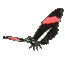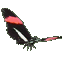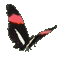Math Help - Name a Line Game - Use Slope & Y-intercept of Graph Tips:

- The line y=4x+5 has a slope of 4 and intersects the y-axis at 5.
- The line y=-1x+0 can be written as y=-x, has a slope of -1 and a y-intercept of 0.
- The line y=0x+3 can be written as y=3, has a slope of 0 and a y-intercept of 3.
- The line y=0x+0 can be written as y=0, and is a horizontal line with y-intercept 0.

- The linear relation y=mx+b represents a set of ordered pairs (x,y) passing through the point (0,b).
The b is called the line's y-intercept and the m is called the line's slope =(rise/run) = rate of change.

- Your Game Score is reduced by the number of butterfly hits.

- To slow the game speed repeat tap/click on the word Slider.
- To increase the game speed repeat tap/click on the word Math.
- Speed can also be adjusted with a keyboard's - and + keys.

- Refresh/Reload the web page to restart the game.